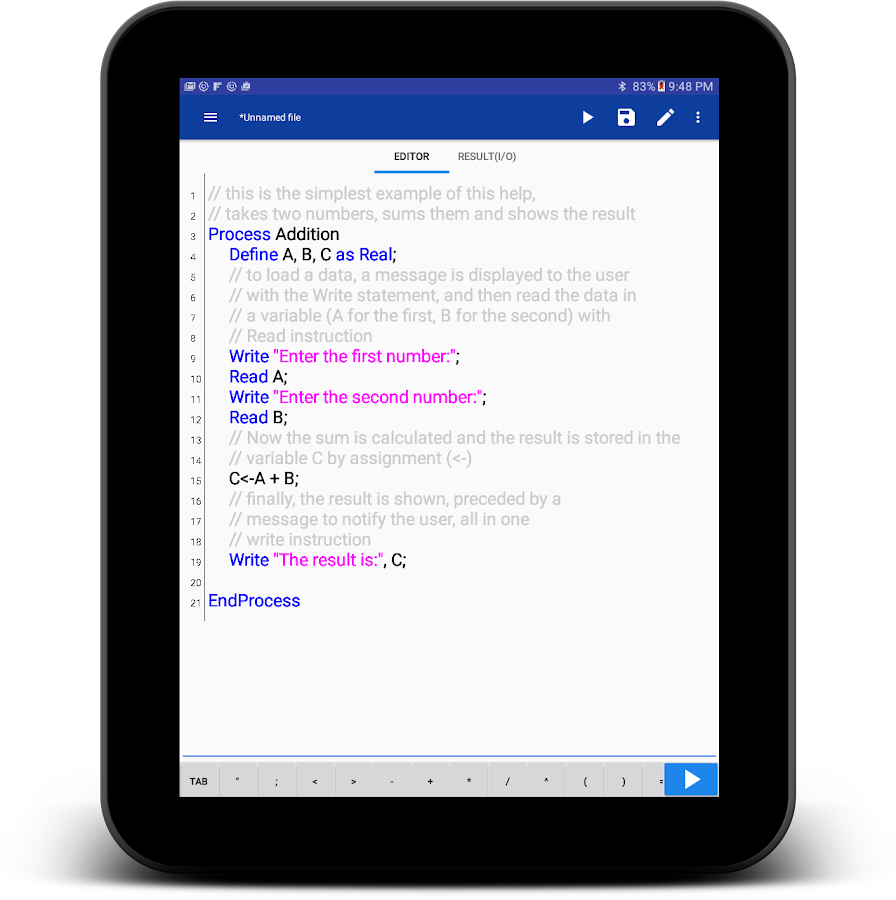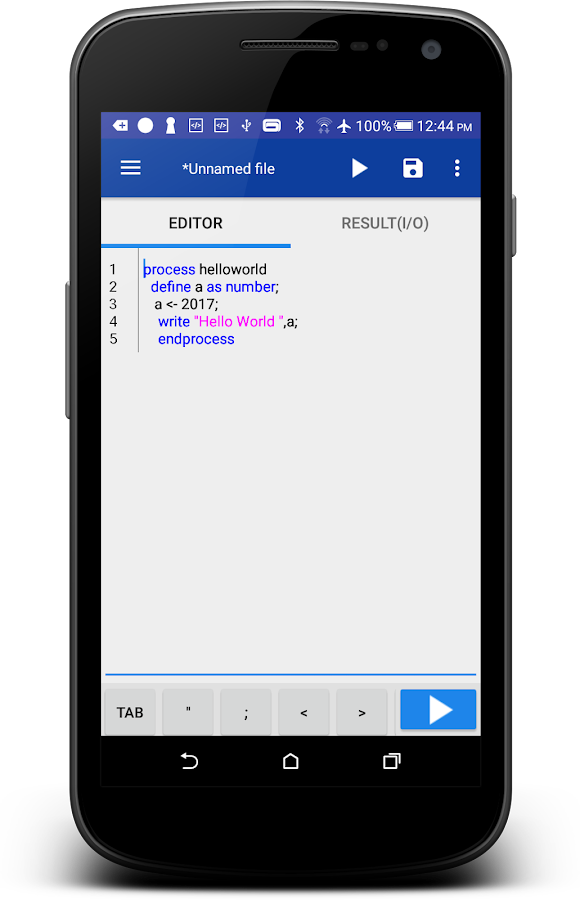DROID-PSECINT PSEudoCode INTerpreter SPN/ENG/... - Printable Version +- HP Forums (https://www.hpmuseum.org/forum) +-- Forum: Not HP Calculators (/forum-7.html) +--- Forum: Not remotely HP Calculators (/forum-9.html) +--- Thread: DROID-PSECINT PSEudoCode INTerpreter SPN/ENG/... (/thread-7492.html) DROID-PSECINT PSEudoCode INTerpreter SPN/ENG/... - compsystems - 12-30-2016 07:32 PM Pseudocode interpreter/(Interprete de seudocódigo), for now only in English and Spanish interpreter, first release version Dec 2016 (Free version) More info ENG: https://play.google.com/store/apps/details?id=pe.diegoveloper.pseudocode&hl=en SPN: https://play.google.com/store/apps/details?id=pe.diegoveloper.pseudocode&hl=es Please test it and comment, Ideas, improvements, etc. are welcome Thanks PD: A friend is the author. If you want to collaborate with the project please translate these two files to a particular language. (French, Portuguese, German, etc.)KEYWORDS.TXT PHP Code: // ENGLISH                                                 // SPANISHBOOL                    : (TRUE | FALSE) ;             // : (VERDADERO | FALSO) ;ALGORITHM               : A L G O R I T H M ;          // : A L G O R I T M O;ENDALGORITHM            : E N D A L G O R I T H M ;    // : F I N A L G O R I T M O;PROCESS                 : P R O C E S S ;              // : P R O C E S O;ENDPROCESS              : E N D P R O C E S S ;        // : F I N P R O C E S O;DEFINE                  : D E F I N E ;                // : D E F I N I R;AS                      : A S ;                        // : C O M O;NUMBER                  : N U M B E R ;                // : N U M E R O;NUMERIC                 : N U M E R I C ;              // : N U M E R I C O;INTEGER                 : I N T E G E R ;              // : E N T E R O;CHARACTER               : C H A R A C T E R ;          // : C A R A C T E R;REAL                    : R E A L ;                    // : R E A L;BOOLEAN                 : B O O L E A N ;              // : L O G I C O;TEXT                    : T E X T ;                    // : T E X T O;STRING                  : S T R I N G ;                // : C A D E N A;READ                    : R E A D ;                    // : L E E R;WRITE                   : W R I T E ;                  // : E S C R I B I R;DIMENSION               : D I M E N S I O N ;          // : D I M E N S I O N;FOR                     : F O R ;                      // : P A R A;TO                      : T O ;                        // : H A S T A;WITH                    : W I T H ;                    // : C O N;STEP                    : S T E P ;                    // : P A S O;DO                      : D O ;                        // : H A C E R;ENDFOR                  : E N D F O R ;                // : F I N P A R A;CLEAR                   : C L E A R ;                  // : B O R R A R;SCREEN                  : S C R E E N ;                // : P A N T A L L A;WAIT                    : W A I T ;                    // : E S P E R A R;KEY                     : K E Y ;                      // : T E C L A;SECONDS                 : S E C O N D S ;              // : S E G U N D O S;SECOND                  : S E C O N D ;                // : S E G U N D O;MILLISECONDS            : M I L L I S E C O N D S ;    // : M I L I S E G U N D O S;IF                      : I F ;                        // : S I;THEN                    : T H E N ;                    // : E N T O N C E S;ELSE                    : E L S E ;                    // : S I N O;ENDIF                   : E N D I F ;                  // : F I N S I;SWITCH                  : S W I T C H ;                // : S E G U N;CASE                    : C A S E ;                    // : C A S O;OF                      : O F ;                        // : D E;ANOTHER                 : A N O T H E R ;              // : O T R O;MODE                    : M O D E ;                    // : M O D O;ENDSWITCH               : E N D S W I T C H ;          // : F I N S E G U N;WHILE                   : W H I L E ;                  // : M I E N T R A S;ENDWHILE                : E N D W H I L E ;            // : F I N M I E N T R A S;REPEAT                  : R E P E A T ;                // : R E P E T I R;THAT                    : T H A T ;                    // : Q U E;SUBPROCESS              : S U B P R O C E S S ;        // : S U B P R O C E S O;ENDSUBPROCESS           : E N D S U B P R O C E S S ;  // : F I N S U B P R O C E S O;FUNCTION                : F U N C T I O N ;            // : F U N C I O N;ENDFUNCTION             : E N D F U N C T I O N ;      // : F I N F U N C I O N;CLEAN                   : C L E A N ;                  // : L I M P I A R;MODULE                  : M O D U L E ;                // : M O D;NO                      : N O ;                        // : N O;TRUE                    : T R U E ;                    // : V E R D A D E R O;FALSE                   : F A L S E ;                  // : F A L S O;SQUAREROOT              : S Q U A R E R O O T ;        // : R C;RANDOM                  : R A N D O M ;                // : A Z A R;  errors.txt PHP Code:     public static final String BOOLEAN_TRUE = "true"; // "verdadero"    public static final BOOLEAN_FALSE = "false"; // "falso"    public static final ERROR_INTEGER = "integer"; // "entero"    public static final ERROR_REAL = "real"; // "real"    public static final ERROR_LOGIC = "logical"; // "logico"    public static final ERROR_STRING = "string"; // "cadena"    public static final ERROR_INVALID_DATA_TYPE = "type not found"; // "tipo no encontrado"    public static final ERROR_INCOMPATIBLE_DATA_TYPE = "incompatible data type. Was expected: "; // "tipos de datos incompatibles. Se esperaba: "    public static final ERROR_WAS_FOUND =";​was found: "; // ";​se encontró: "    public static final ERROR_INVALID_BOOLEAN = "Incorrect Value for Boolean Variable"; // "Valor incorrecto para la variable booleana"    public static final ERROR_INVALID_TYPE = "invalid data type"; // "tipo de dato inválido"    public static final ERROR_SYMBOL_NAME_1 = "the symbol with name \""; // "el simbolo con nombre \""    public static final ERROR_SYMBOL_NAME_2 = "\" has already been declared "; // "\" ya ha sido declarado"     public static final ERROR_INTEGER_OR_REAL = "integer or real"; // "entero o real"    public static final ERROR_ARITHMETIC_NEGATION = "error in arithmetic negation"; // "error en negación aritmética"    public static final ERROR_BOOLEAN_NEGATION = "error in Boolean negation"; // "error en negación booleana"    public static final ERROR_RETURN_VAR_CREATED = "return variable was not created"; // "la variable de retorno no fue creada"    public static final ERROR_RETURN_VAR_INITIALIZED = "return variable not initialized"; // "variable de retorno no fue inicializada"    public static final ERROR_EXECUTION_CANCELED = "Execution canceled"; // "Ejecución cancelada"    public static final ERROR_INVALID_PARAMETERS = "Input parameters are missing"; // "Faltan parámetros de entrada"    public static final ERROR_INVALID_VARIABLE = "Unidentified variable"; // "Variable no identificada"    public static final ERROR_ARRAY_VARIABLE_1 = "the variable \""; // "la variable \""    public static final ERROR_ARRAY_VARIABLE_2 = "\" already initialized as not array"; // "\" ya se inicializo como no arreglo"    public static final ERROR_ARRAY_VARIABLE_3 = "can not declare that value in the array"; // "no se puede declarar ese valor en el arreglo"    public static final ERROR_DIVISION_BY_ZERO = "division between 0"; // "division entre 0"    public static final ERROR_INVALID_OPERATOR = "unknown operator"; // "operador desconocido"    public static final ERROR_SEMANTIC = "Semantic error"; // "Error semántico"    public static final ERROR_RUNTIME = "Runtime Error"; // "Error en tiempo de ejecución"    public static final ERROR_FUNCTION_WITH_NAME_0 = "the function \""; // "la función \""    public static final ERROR_FUNCTION_WITH_NAME_1 = "the function with name\""; // "la función con nombre \""    public static final ERROR_FUNCTION_WITH_NAME_2 = "\" has not been declared"; // "\" no ha sido declarada"    public static final ERROR_FUNCTION_WITH_NAME_3 = "\" returns a value that must go in an expression"; // "\" retorna un valor que debe ir en una expresión"    public static final ERROR_FUNCTION_WITH_NAME_4 = "\" is used in an expression but returns no value"; // "\" es usada en una expresión pero no retorna ningún valor"    public static final ERROR_FUNCTION_WITH_NAME_5 = "número incorrecto de parametros al llamar la función \""; // "número incorrecto de parametros al llamar la función \""    public static final ERROR_VARIABLE_WITH_NAME_1 = "the variable named \""; // "la variable con nombre \""    public static final ERROR_VARIABLE_WITH_NAME_2 = "\" has not been initialized"; // "\" no ha sido inicializada"    public static final ERROR_INVALID_INDEX_1 = "The index \""; // "El índice \""    public static final ERROR_INVALID_INDEX_2 = "\" in the array \""; // "\" en el arreglo \""    public static final ERROR_INVALID_INDEX_3 = "\" it's not valid"; // "\" no es válido"    public static final ERROR_INVALID_INDEX_4 = "an invalid position of the array was accessed:"; // "se accedió a una posición no valida del arreglo: "    public static final ERROR_INVALID_SYMBOL_1 = "the symbol with name \""; // "el símbolo con nombre \""    public static final ERROR_INVALID_SYMBOL_2 = "has already been declared \""; // "ya ha sido declarado \""    public static final ERROR_INVALID_DATA = "Incorrect data"; // "Datos incorrectos"  Future release is to compete with the PC version (http://pseint.sourceforge.net/index.php?page=descargas.php), which includes: 1: Step by Step Execution 2: Generate flowcharts 3: export pseudocode to n computer languages (c++/java/matlab, .... etc)